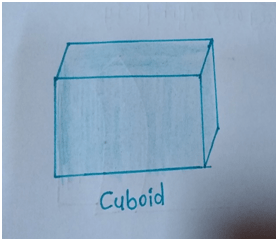# Faces, edges and vertices

In geometry, we see different objects which are in 2D shapes and some are in 3D shapes also. The geometric shapes like square, rectangle, circle, triangle are the two dimensional objects which are having sides and vertices only.

But there are some 3D shapes like sphere, cube, cone, cylinder, tetrahedron etc are the 3 dimensional objects which are having faces, edges and vertices also.

On the basis of their geometrical shapes they are having different properties.

We can identify any 3D object without seeing it, by only from its faces, edges and vertices configuration.

There is also a relationship between number of faces, number of edges and number of vertices on the basis of which we decide what the geometrical shape of the given object is also.

The following are the fundamental properties of 3D objects which are explained in detail.

### Faces:

Every 3D object is having flat surface, the individual flat surface which cannot be divided in other part is the face of the object.

Faces are the planes in two dimensions.

### Edges:

The two faces of the 3D objects meets each other along a particular line which is called as edge of that object.

The edges of the objects form its skeleton in three dimensions.

### Vertices:

The vertex is the point where two or more than two edges meet each other. The two dimensional objects has vertices and edges also.

Following are the some three dimensional objects described with their geometrical properties.

#### 1) Cuboid:

The following figure shows the cuboid, which is having length, breadth and height in different measure.

The cuboid has total 6 faces, 12 edges and 8 vertices as shown in figure.#### 2) Cube:

Cube is the special type of cuboid which is having length, breadth and height all in equal measure.

The following figure shows the cube.

It has total 6 faces, 12 edges and 8 vertices similar to the cuboid, as shown in figure below.#### 3) Sphere:

Sphere is the three dimensional geometrical figure having surface area with specific radius as shown in fig.

The sphere has one curved surface only throughout.

Hence, sphere has only one face, and only one edge with no vertices as shown in figure.#### 4) Cylinder:

It is the three dimensional geometrical shape having length, radius and two flat surfaces which are circular and one curved surface as shown in figure.

Thus, cylinder has 3 faces, 2 edges and zero vertices as shown in figure.#### 5) Cone:

Cone is the three dimensional geometrical figure which is having flat circular surface which is ended at other end in only one vertex as shown in figure.

Thus, cone has 2 faces one is curved and other is flat circular face as shown in figure.

It has only one edge and only one vertex as shown in figure.#### 6) Tetrahedron:

The following is three dimensional geometrical figure which shows the tetrahedron.

It has total 4 faces, 6 edges and 4 vertices as shown in figure.#### 7) Triangular prism:

The following three dimensional geometrical figure shows the Triangular prism.

It has total 5 faces, 9 edges and 6 vertices as shown in figure below.#### 8) Square based pyramid:

The following figure shows the three dimensional geometrical figure which is the square based pyramid.

It has the base which is in the shape like square.

It has total 5 faces, 8 edges and 5 vertices as shown in figure below.### Euler’s formula:

Euler’s formula gives the relationship between faces, edges and vertices of the three dimensional geometrical shapes which are having only flat surfaces like cuboid, cube etc.

For the three dimensional geometrical shapes having curved surface like sphere, cone, cylinder etc. we can’t apply the Euler’s formula.

It gives the mathematical relation as,

F + V – E = 2

Where F means number of faces, V means number of vertices and E means number of edges.

For example:

We check for shape cube, it satisfy the Euler’s formula or not.

• Cube has 6 faces, 12 edges and 8 vertices.

Hence, F= 6, V = 8 and E = 12.

Thus, Euler’s formula becomes,

F + V – E = 6 + 8 – 12 = 14 – 12 = 2

Thus, Euler’s relation is satisfied by the cube.

• Similarly, we check Euler’s formula for tetrahedron.

Tetrahedron has 4 faces, 6 edges and 4 vertices.

Hence, F = 4, E= 6, V = 4.

Thus, Euler’s formula becomes,

F + V – E = 4 + 4 – 6 = 8 – 6 = 2

Thus, Euler’s relation is satisfied by the tetrahedron.

Updated: July 22, 2021 — 7:36 pm